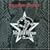# Forum

## GL_OrthoMatrix (RMQ engine style)

Discuss programming topics that involve the OpenGL API.

Moderator: InsideQC Admins

### GL_OrthoMatrix (RMQ engine style)

Calc ortho matrix4x4 in a manner similar to MH's matrix functions in RMQ engine. Which are like a work of art.

And make the other source codes I've seen floating around on the internet for the same functions look a bit messy.

Code: Select all
`glmatrix *GL_OrthoMatrix (glmatrix *m, float left, float right, float bottom, float top, float near, float far){      float r_l = right - left;    float t_b = top - bottom;    float f_n = far - near;    float tx = - (right + left) / (right - left);    float ty = - (top + bottom) / (top - bottom);    float tz = - (far + near) / (far - near);   glmatrix tmp;   GL_IdentityMatrix (&tmp);    tmp.m16 = 2.0f / r_l;    tmp.m16 = 0.0f;    tmp.m16 = 0.0f;    tmp.m16 = tx;    tmp.m16 = 0.0f;    tmp.m16 = 2.0f / t_b;    tmp.m16 = 0.0f;    tmp.m16 = ty;    tmp.m16 = 0.0f;    tmp.m16 = 0.0f;    tmp.m16 = 2.0f / f_n;    tmp.m16 = tz;    tmp.m16 = 0.0f;    tmp.m16 = 0.0f;    tmp.m16 = 0.0f;    tmp.m16 = 1.0f;      GL_MultiplyMatrix (m, &tmp, m);   return m;}`

Using the calcs from this:

Untested at the moment. Will be updated should it need adjustment.
The night is young. How else can I annoy the world before sunsrise?Inquisitive minds want to know ! And if they don't -- well like that ever has stopped me before ..Baker

Posts: 3666
Joined: Tue Mar 14, 2006 5:15 am

### Re: GL_OrthoMatrix (RMQ engine style)

They're heavily based on the D3DX matrix functions (which I use in my experimental Q2 engine and which is otherwise GL).
We had the power, we had the space, we had a sense of time and place
We knew the words, we knew the score, we knew what we were fighting formh

Posts: 2292
Joined: Sat Jan 12, 2008 1:38 am

### Re: GL_OrthoMatrix (RMQ engine style)

The above isn't "right" because it has the identity matrix "baked in".

That might be a little more efficient, but doesn't actually do the same thing as glOrtho as result.

Replacement:

Code: Select all
`glmatrix *GL_OrthoMatrix (glmatrix *m, float left, float right, float bottom, float top, float znear, float zfar){      glmatrix tmp;   float rml = right - left;   float fmn = zfar - znear;   float tmb = top - bottom;   float _1over_rml, _1over_fmn, _1over_tmb;#ifdef TESTING   float check;   glMatrixMode (GL_PROJECTION);   glLoadIdentity ();   glOrtho (left, right, top, bottom, znear, zfar);   glGetFloatv (GL_PROJECTION_MATRIX, check);#endif      if (rml == 0.0 || fmn == 0.0 || tmb == 0.0)    {      // Divide by 0 error condition      return NULL;   }   _1over_rml = 1.0f / rml;   _1over_fmn = 1.0f / fmn;   _1over_tmb = 1.0f / tmb;   tmp.m16 = 2.0f * _1over_rml;   tmp.m16 = 0.0f;   tmp.m16 = 0.0f;   tmp.m16 = 0.0f;   tmp.m16 = 0.0f;   tmp.m16 = 2.0f * _1over_tmb;   tmp.m16 = 0.0f;   tmp.m16 = 0.0f;   tmp.m16 = 0.0f;   tmp.m16 = 0.0f;   tmp.m16 = -2.0f * _1over_fmn;   tmp.m16 = 0.0f;   tmp.m16 = -(right + left) *  _1over_rml;   tmp.m16 = -(top + bottom) *  _1over_tmb;   tmp.m16 = -(zfar + znear) * _1over_fmn;   tmp.m16 = 1.0f;   GL_MultiplyMatrix (m, &tmp, m);   return m;}`

Above code a modification of : this

mh wrote:They're heavily based on the D3DX matrix functions (which I use in my experimental Q2 engine and which is otherwise GL).

I like 'em.I don't like having to pop the matrix or restore gl states, so I'm kinda scrubbing that stuff out entirely ...
The night is young. How else can I annoy the world before sunsrise?Inquisitive minds want to know ! And if they don't -- well like that ever has stopped me before ..Baker

Posts: 3666
Joined: Tue Mar 14, 2006 5:15 am

### Re: GL_OrthoMatrix (RMQ engine style)

shouldn't really need a matrix multiply within that function at all. the calculated matrix IS the projection matrix.
the only way the multiply could possibly be useful is texture projection or glsl (where modelviewproj can be provided directly).
Spike

Posts: 2894
Joined: Fri Nov 05, 2004 3:12 am
Location: UK

### Re: GL_OrthoMatrix (RMQ engine style)

Spike wrote:shouldn't really need a matrix multiply within that function at all. the calculated matrix IS the projection matrix.
the only way the multiply could possibly be useful is texture projection or glsl (where modelviewproj can be provided directly).

I ended up realizing that after thinking it over (re: the very first part, the second part I knew .. then again based on a comment above, maybe it seems like I didn't knowBut rather I had my brain turned off and it didn't click at that moment ...).

I know most of the matrix concepts in the abstract, and slowly getting a handle on them in actual internal matrix calculations side. So when I'm looking at the matrix calculations themselves like above, yeah it doesn't always register immediately.I would say the growing pains of learning this stuff sucks. Except it doesn't.

For instance, when I saw MH's tutorial on proper dynamic lighting, I already knew what I was going to see in concept (either the light or the entity matrix translated/rotated), but understanding something in theory doesn't mean you can do something in practice ... at least not time efficiently
The night is young. How else can I annoy the world before sunsrise?Inquisitive minds want to know ! And if they don't -- well like that ever has stopped me before ..Baker

Posts: 3666
Joined: Tue Mar 14, 2006 5:15 am

Return to OpenGL Programming

### Who is online

Users browsing this forum: No registered users and 1 guest